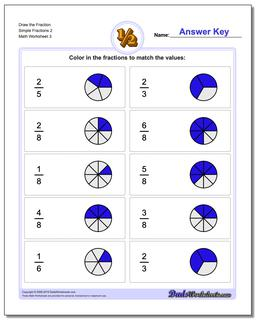# Math Worksheets: Graphic Fractions: Graphic Fractions: Draw the Fraction Simple Fractions 2 (Third Worksheet)## Draw the Fraction Simple Fractions 2 (Third Worksheet)

PropertyValue
DescriptionDraw the Fraction Simple Fractions 2: These beginning problems allow kids to practice showing a fraction as a mixed value. These worksheets provide help in the form of pie charts pre-divided based on the divisor with undivided shapes to represent the wholes where appropriate. (Third Worksheet)
Resource TypeWorksheet